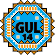General Utility Library for C++14  2.7
Statistics Utilities

The General Utility Library offers some utilities to calculate statistics on data in arbitrary containers.

All of the functions are templated and allow specifying an optional accessor function so that they can be used on practically any data type.

### Functions

accumulate(): Return the values of all elements somehow combined. A closure has to be specified to describe how to values are combined.

maximum(): Return the maximum value.

mean(): Calculate the arithmetic mean.

median(): Calculate the median.

minimum(): Return the minimum value.

min_max(): Return the minimum and maximum value.

remove_outliers(): Remove the data points that are the furthest from the mean of all data points. If more than one point is to be removed this is done recursively with intermediate recalculations of the mean.

rms(): Calculate the root-mean-square value.

standard_deviation(): Calculate the standard deviation.

### Classes

MinMax: Holds a pair of two values, typically the minimum and maximum element of something.

StandardDeviationMean: Holds a pair of two values, typically the standard deviation and the mean value of something.